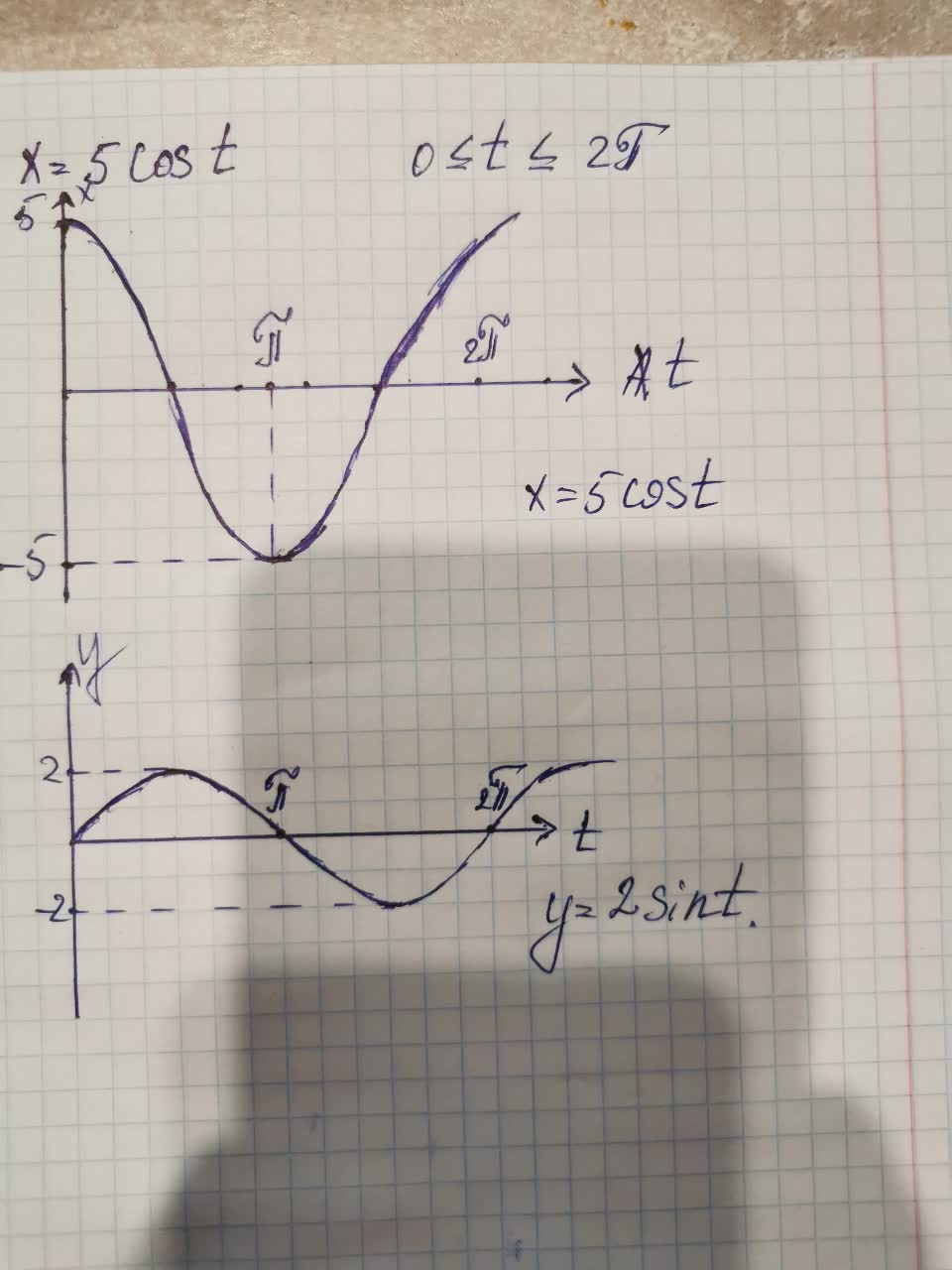# Sketch the parametric curve for the following set of parametric equations. Clearly indicate direction of motion 1)x=5cost 2)y=2sint 3)0>=t>=2piwaigaK 2021-09-04 Answered
Sketch the parametric curve for the following set of parametric equations. Clearly indicate direction of motion
1)$x=5\mathrm{cos}t$
2)$y=2\mathrm{sin}t$
3)$0\ge t\ge 2\pi$
You can still ask an expert for help

• Questions are typically answered in as fast as 30 minutes

Solve your problem for the price of one coffee

• Math expert for every subject
• Pay only if we can solve itTheodore Schwartz

To sketch the parametric curve for the following set of parametric equations you need